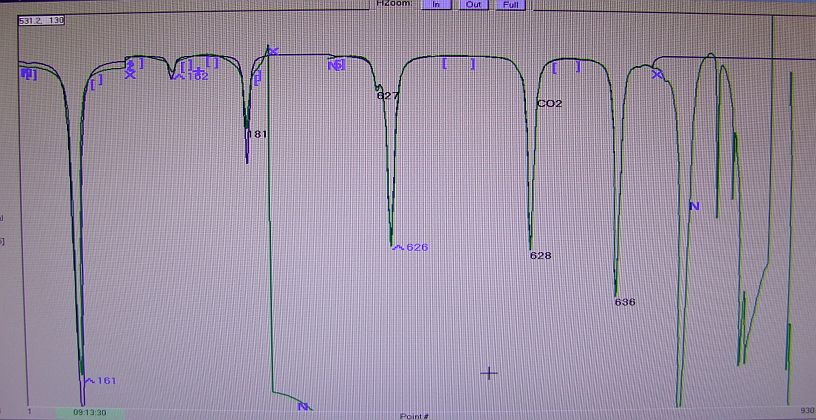# Data corrections – IntroductionThis display from the TDLWintel software shows the absorption responses for all the CO2 and water isotopologues

Stable isotope ratio mass spectrometers return all their analytical values as ratios: for example the carbon isotope ratio of carbon dioxide is basically expressed as mass 45/mass 44, with a natural abundance correction such as the Craig correction.  Ratios are used because the sources are relatively unstable in time but at any given instant the ratio of one beam to another is relatively correct.  This is not so much true for lasers where temporal stability is much better.  So the results are expressed as concentrations: light is absorbed by a molecule vibrating at the frequency of the lased light.  The absorption (described by the Beer-Lambert law) is determined essentially by the absorption cross-section of the material, its concentration and how far the beam has to travel through it.  These are all constants in a given machine apart from the concentration.  But note that there are complications: for example the absorption is measured in the cell, but there is also some CO2 in the path that the laser follows to the cell.  As this latter absorption does not vary with the analyte concentration IN the cell, this alone will result in a non-linearity.

The laser is held had a carefully controlled temperature by Peltier cooling or similar.  The laser current is then rapidly cycled to cause the laser frequency to scan the frequencies of interest – many times every second.  A visit to the HITRAN database site will show that each isotopic variant of CO2 (each isotopologue) absorbs at many wavelengths.  The frequencies chosen may not be the ones that are the most sensitive for measurement – electrical constraints on the laser detectors mean that it is more important to choose frequencies where the total proportion of absorption is similar, even though the isotopologue abundances may be very different.  In addition the laser can only scan over a limited range so the selected frequencies have to be fairly close together and, at present, the same laser cannot scan water and carbon dioxide.

So what isotopologues are we looking at?  For carbon dioxide these are 626 (16O12C16O), 627 (17O12C16O), 628 (18O12C16O) and 636 (16O13C16O).  Of course there are others, such as 638 (16O13C18O) but by assuming statistical distributions (as the Craig correction does) the errors induced by not measuring them are extremely small.  The HITRAN database gives abundances for the different isotopologues in the atmosphere and the numbers returned by the Aerodyne are scaled by these.  So what does TDLWintel, the Aerodyne software, return?  As an example, if you fed in CO2 with a VPDB composition of 0 permil and a concentration of 400 ppm into the QCL, the software would return: 626 = 400, 627 = 400, 628 = 400 and 636 = 400.  (Actually it returns them in ppb but we’ll pretend that it’s ppm).  So if we want to see the actual ppm of 628 (carbon dioxide with a light carbon and an O-18) then we go to the HITRAN data and find that they give an abundance for 628 of 0.003947.  If we multiply the 400 ppm by that we get 1.5788 ppm or not very much!  Embedded in this number are of course the corrections for path length plus absorption cross-section plus temperature plus detector gain etc.  The temperature function is very steep and needs to be quite precise!  So the temperature of the laser cabinet must be very stable.  As the electronics also respond to temperature change, the instrument requires a stable environment to give its best performance.

Another issue to be considered is band broadening: the gas molecules interact with each other and interact more with molecules of a similar vibrational frequency.  So the gas mix affects the analysis.  While the peak area remains the same, the amplitude decreases and the width increases with broadening, so accurate integration of the whole absorption spectrum is essential.  TDLWintel takes care of this task.  The amount of carbon dioxide present and its isotopic composition should therefore influence the analysis, but we are assured that the magnitude of this effect is tiny.   This is not true for oxygen, even though it is a “Foreign gas” and has dissimilar vibrations.  Why?  Because carbon dioxide is typically around 400 ppm while oxygen is typically around 210,000 ppm!  Even on a coarse scale it is imperative to calibrate for the oxygen proportion.  We also find a strong effect for water vapour.  TDLWintel software includes a function for compensating for water broadening but we have not experimented with it.

Page 2 – thinking about isotope values